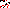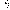# MINLPLib

### A Library of Mixed-Integer and Continuous Nonlinear Programming Instances

#### Instance st_e03

 Formatsⓘ ams gms mod nl osil pip py Primal Bounds (infeas ≤ 1e-08)ⓘ -1161.33660200 p1 ( gdx sol ) (infeas: 2e-09) Other points (infeas > 1e-08)ⓘ Dual Boundsⓘ -1161.33660400 (ANTIGONE)-1161.33660400 (BARON)-1161.33660200 (COUENNE)-1161.33660200 (LINDO)-1161.33660300 (SCIP) Referencesⓘ Bracken, Jerome and McCormick, Garth P, Selected Applications of Nonlinear Programming, John Wiley and Sons, New York, 1968.Tawarmalani, M and Sahinidis, N V, Convexification and Global Optimization in Continuous and Mixed-Integer Nonlinear Programming: Theory, Algorithms, Software, and Applications, Kluwer, 2002. Sourceⓘ BARON book instance misc/e03 Added to libraryⓘ 03 Sep 2002 Problem typeⓘ NLP #Variablesⓘ 10 #Binary Variablesⓘ 0 #Integer Variablesⓘ 0 #Nonlinear Variablesⓘ 7 #Nonlinear Binary Variablesⓘ 0 #Nonlinear Integer Variablesⓘ 0 Objective Senseⓘ min Objective typeⓘ quadratic Objective curvatureⓘ indefinite #Nonzeros in Objectiveⓘ 6 #Nonlinear Nonzeros in Objectiveⓘ 2 #Constraintsⓘ 7 #Linear Constraintsⓘ 3 #Quadratic Constraintsⓘ 2 #Polynomial Constraintsⓘ 2 #Signomial Constraintsⓘ 0 #General Nonlinear Constraintsⓘ 0 Operands in Gen. Nonlin. Functionsⓘ Constraints curvatureⓘ indefinite #Nonzeros in Jacobianⓘ 21 #Nonlinear Nonzeros in Jacobianⓘ 9 #Nonzeros in (Upper-Left) Hessian of Lagrangianⓘ 13 #Nonzeros in Diagonal of Hessian of Lagrangianⓘ 1 #Blocks in Hessian of Lagrangianⓘ 2 Minimal blocksize in Hessian of Lagrangianⓘ 2 Maximal blocksize in Hessian of Lagrangianⓘ 5 Average blocksize in Hessian of Lagrangianⓘ 3.5 #Semicontinuitiesⓘ 0 #Nonlinear Semicontinuitiesⓘ 0 #SOS type 1ⓘ 0 #SOS type 2ⓘ 0 Minimal coefficientⓘ 6.6700e-03 Maximal coefficientⓘ 9.8000e+04 Infeasibility of initial pointⓘ 102 Sparsity JacobianⓘSparsity Hessian of Lagrangianⓘ```\$offlisting
*
*  Equation counts
*      Total        E        G        L        N        X        C        B
*          8        7        1        0        0        0        0        0
*
*  Variable counts
*                   x        b        i      s1s      s2s       sc       si
*      Total     cont   binary  integer     sos1     sos2    scont     sint
*         11       11        0        0        0        0        0        0
*  FX      0
*
*  Nonzero counts
*      Total    const       NL      DLL
*         28       17       11        0
*
*  Solve m using NLP minimizing objvar;

Variables  x1,x2,x3,x4,x5,x6,x7,x8,x9,x10,objvar;

Positive Variables  x3,x5;

Equations  e1,e2,e3,e4,e5,e6,e7,e8;

e1..    x1 - 1.22*x4 + x5 =E= 0;

e2..    x9 + 0.222*x10 =E= 35.82;

e3..    3*x7 - x10 =E= 133;

e4.. 0.038*sqr(x8) - 1.098*x8 - 0.325*x6 + x7 =E= 57.425;

e5.. x4*x9*x6 + 1000*x3*x6 - 98000*x3 =E= 0;

e6.. -x1*x8 + x2 + x5 =E= 0;

e7.. 0.13167*x8*x1 + 1.12*x1 - 0.00667*sqr(x8)*x1 - x4 =G= 0;

e8.. 0.063*x4*x7 - 5.04*x1 - 0.035*x2 - 10*x3 - 3.36*x5 + objvar =E= 0;

* set non-default bounds
x1.lo = 1; x1.up = 2000;
x2.lo = 1; x2.up = 16000;
x3.up = 120;
x4.lo = 1; x4.up = 5000;
x5.up = 2000;
x6.lo = 85; x6.up = 93;
x7.lo = 90; x7.up = 95;
x8.lo = 3; x8.up = 12;
x9.lo = 1.2; x9.up = 4;
x10.lo = 145; x10.up = 162;

Model m / all /;

m.limrow=0; m.limcol=0;
m.tolproj=0.0;

\$if NOT '%gams.u1%' == '' \$include '%gams.u1%'

\$if not set NLP \$set NLP NLP
Solve m using %NLP% minimizing objvar;

```

Last updated: 2023-06-01 Git hash: fdbb0df7